# FLARE On Challenge (2015) #11

This is the final challenge and it is said to be more difficult than the previous challenges. The file of this challenge named CryptoGraph, which is a 32 bit Windows Portable Executable file.

Let’s analyze it in IDA at first. The flow of the main() function looks like below: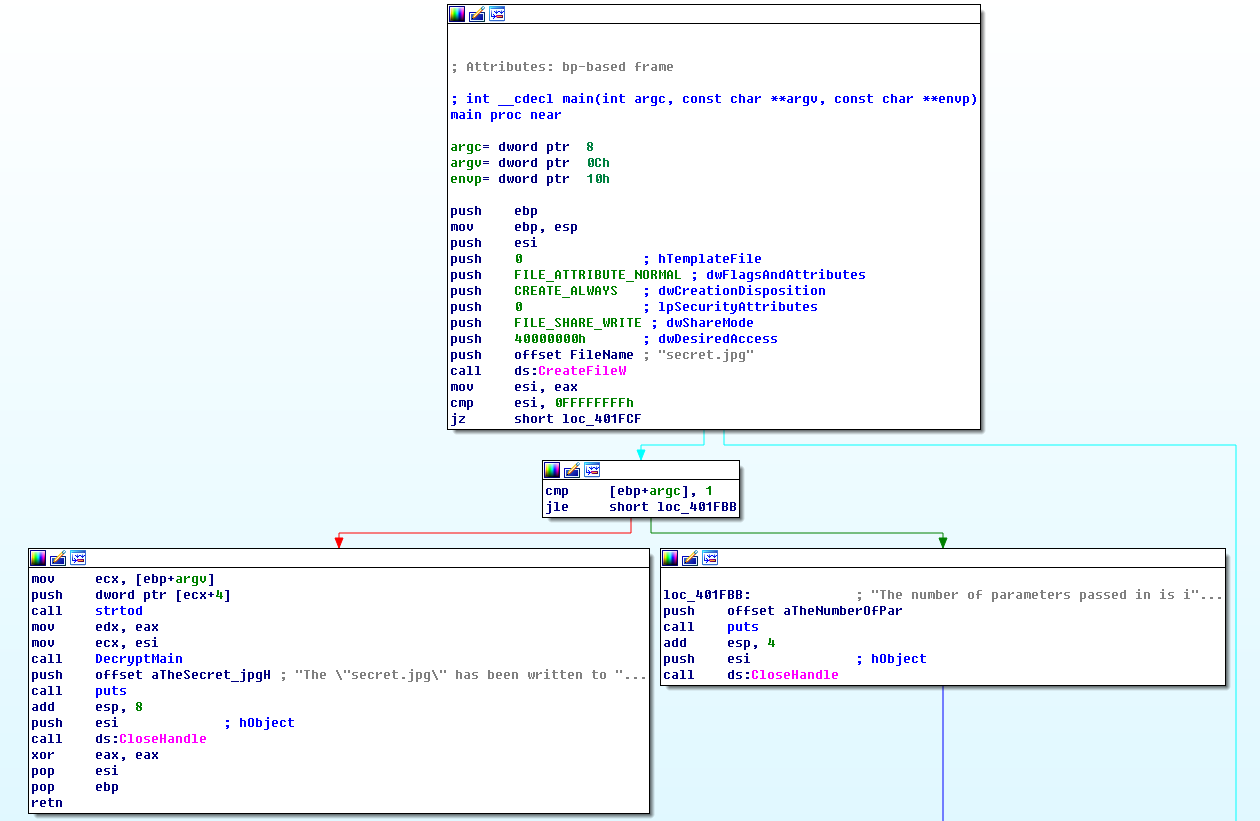The main() function of this program does some simple works: it creates a file named secret.jpg, then checks if the number of the arguments is more than 1, if so it will convert the second argument to an DWORD and decrypt some data into secret.jpg using this DWORD as a “key”. Since the second argument is the only input used to decrypt the jpg file, I call it Masterkey. And if the number of the arguments equals to or less than 1, it will print out the following message:
`The number of parameters passed in is incorrect.`

The function decrypts the jpg file is located at address 0x00401910 (shown as DecryptMain() in above picture), this function takes two parameters passed through register edx and ecx, they are the Masterkey and the file handle of the secret.jpg respectively.

At the beginning of function DecryptMain(), it loads and verifies the size of two resources whose id is 120 and 121. The picture below shows a piece of code that loads the resource 120 and verifies if it size is larger than 0x30 bytes: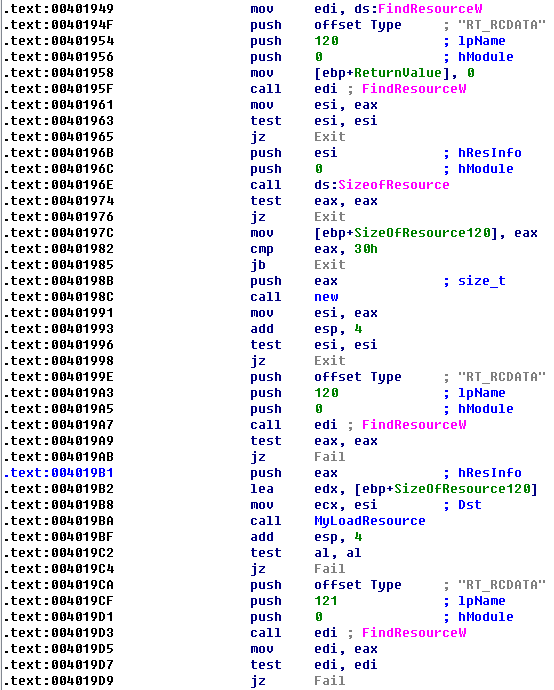After loads the two resources, it will initialize a class which I named it as CryptoInfo. The structure of this class is shown as below: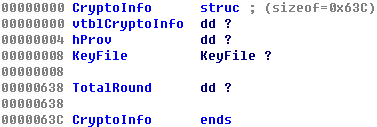The virtual table (vtblCryptoInfo) of this class contains two functions GenRandom() and IsInit():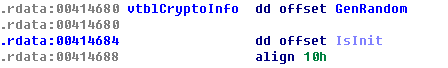The hProv of this class is a handle of the Cryptographic Service Provider (CSP) obtained by calling API CryptAcquireContextW(), and the KeyFile and TotalRound will be described later in this article.

Next, a function located at address 0x00401A81 (the CalcDecryptKey() function in the picture below) will be used to calculate the KeyBlocks (will describe later), and function located at address 0x00401A99 (the DecryptImage() function in the picture below) will be used to decrypt the final data and save it to the jpg file: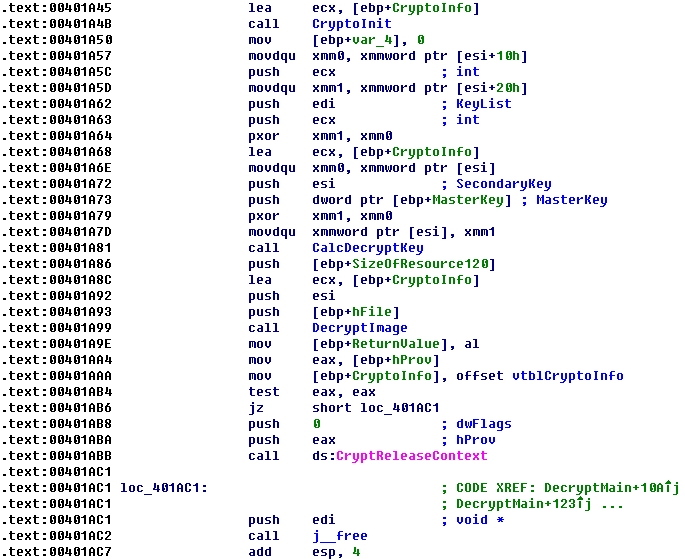The CalcDecryptKey() function takes three parameters: the MasterKey, the SecondaryKey and the resource 121. The SecondaryKey is calculated from resource 120 by XORing each 16 byte blocks and it will be used to decrypt the first KeyBlock (will describe later) in resource 121. The resource 121 contains a list of keys which will be used to decrypt the final data, so I call it KeyFile. The KeyFile has the following structure: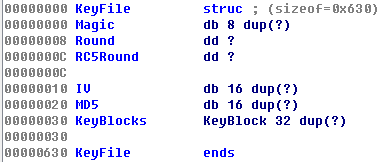The Magic field in the KeyFile structure is an ASCII string “FLARE-ON”. The Round, RC5Round and IV are used by the decryption algorithms of this program, and each of them will be described in details later. The MD5 is the hash checksum of the KeyBlocks, and the KeyBlocks is an array of a structure named KeyBlock which contains the key to decrypt the final data.

In the CalcDecryptKey() function, the KeyFile is verified by following steps at first:
1. If there is a magic string “FLARE-ON” at the beginning of the KeyFile;
2. If the MD5 hash of the KeyBlocks (from offset 0x30 to offset 0x630) matches the MD5 hash (at offset 0x20) of the KeyFile.

After verifying the KeyFile, a RC5Key is calculated based on the SecondaryKey and the 16 bytes initiate vector (IV) located at offset 0x10 of the KeyFile. And the MasterKey will serve as the first byte of the IV, which indicates that the MasterKey must be a value between 0 and 255.

This program uses an algorithm which I am not familiar with to calculate the RC5key, I have translated the algorithm into following Python code:

```def generate_key(key, iv):
buffer1 = key + '\x00' * 48
buffer2 = key + '\x00' * 48

buffer1 = str(bytearray((ord(ch) ^ 0x36) for ch in buffer1))
buffer2 = str(bytearray((ord(ch) ^ 0x5c) for ch in buffer2))

iv_md5 = calc_md5_ex(buffer1, iv)

buffer2 += iv_md5
key = calc_md5(buffer2)

return key

def generate_rc5key(secondarykey, iv, round):
rc5key = ''

secondarykey_md5 = calc_md5(secondarykey)
rc5key = generate_key(secondarykey_md5, iv + '\x00\x00\x00\x01')

for i in range(0, round - 1):
tmpkey = generate_key(secondarykey_md5, rc5key)
rc5key = str(bytearray((ord(tmpkey[i]) ^ ord(rc5key[i])) for i in range(0, 16)))

return rc5key
```

The function generate_rc5key() in the script shows how this program generate the RC5Key, the parameter secondarykey has been described before — it is calculated from resource 120 by XORing each 16 byte blocks; the iv comes from offset 0x10 of the KeyFile structure and the round comes from offset 0x08 of the KeyFile structure.

Next, the RC5Key is used to decrypt the first KeyBlock in the KeyFile (at offset 0x30) with a slightly modified version of RC5 algorithm, this algorithm will XOR the first block (note: one block is 8 bytes) of the original RC5 decrypted data with an index value and XOR the followed blocks of the RC5 decrypted result with the encrypted data. The following Python code imitates how this algorithm works:

```from Crypto.Cipher import RC5

def rc5_decrypt(key, data, round, index):
rc5cipher = RC5.new(rc5key, mode = RC5.MODE_ECB, rounds = round)
rc5_data = rc5cipher.decrypt(data)

block1 = struct.pack('<I', index) * 2
block2 = rc5_data[0x00:0x08]
dec_data = str(bytearray((ord(block1[x]) ^ ord(block2[x])) for x in range(0, 0x08)))

block1 = data[0x00:0x28]
block2 = rc5_data[0x08:0x30]
dec_data += str(bytearray((ord(block1[x]) ^ ord(block2[x])) for x in range(0, 0x28)))

return dec_data
```

The decrypted KeyBlock has the following structure: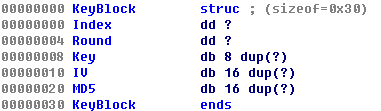The Index is a numeric value count from 0. The Round and IV are used to decrypt the next KeyBlock. The Key will be used to decrypt the final data. And the MD5 is the hash checksum of the first 0x20 bytes of the KeyBlock structure.

After decrypted the first KeyBlock, we can continue to decrypt the second KeyBlock by using the MD5 hash of the Key in the first KeyBlock as NewSecondaryKey and the IV in the first KeyBlock as NewIV. The NewRound is calculated based on the old round value and the Round field in the first KeyBlock. Then sample algorithm (see the generate_rc5key() function described before) is used to calculate a NewRC5Key and the second KeyBlock is decrypted with this NewRC5Key.

Repeat the above steps we can theoretically decrypt all the 32 KeyBlocks and once we have all the KeyBlocks, we may decrypt the jpg file.

So our task become clearly: we need to find out the correct MasterKey so that we can decrypt all the KeyBlocks. Till now we have the following information:
1. The Masterkey is an integer between 0 and 255 and it serves as the first byte of the IV which is used to calculate the RC5Key.
2. The RC5Key is used to decrypt the first KeyBlock.
3. The first KeyBlock has an Index and a MD5 checksum which can be used to verify itself.

With above knowledge, we can do a brute force attack on the Masterkey by checking if the Index field of the first decrypted KeyBlock equals to 0.

This could be done with many approaches. One easiest way is to modify the first instruction of the code that only can be executed when the Index of the first KeyBlock is correct to a software breakpoint (int 3, or 0xCC), and call the modified program with argument from 0 to 255 to see which one will crash the program.

But here I use a WinDbg script to do the same thing, the script is as below:

```import pykd
import time

g_index = 0

def bp_callback(event):
global g_index
out = ''
out += chr(b)
open('D:\\Work\\out.txt', 'ab').write(str(g_index) + ': ' + out.encode('hex')+'\r\n')
print out.encode('hex')
return True

for i in range(200, 256):
g_index = i
pykd.startProcess('D:\\Work\\CryptoGraph.exe ' + str(i))
pykd.setBp(0x004016D4, bp_callback)
pykd.go()
time.sleep(1)
pykd.dbgCommand('bc *')
pykd.killProcess
```

The script does the following tasks:
1. Run process CryptoGraph.exe with different augments (from 0 to 255).
2. Set a breakpoint at address 0x004016D4, right after the program decrypted the first KeyBlock. If the program stopped at this address, the register esi will point to the decrypted data.
3. Dump the decrypted data into a file.

Executed this script in WinDbg and from the output file we can easily find the correct MasterKey: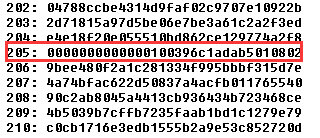Now that we have the MasterKey, we can decrypt all the KeyBlocks and then decrypt the final data to get the email address.

Unfortunately, things not that easy. As we have described before, the NewRound value used the calculated the next RC5Key is based on the old round value and the Round filed in the previous KeyBlock, however, the increase of this value may lead to several hours or even several days to decrypt all the KeyBlocks, because it determines how many loops we should go through.

With the belief that the challenge should not aim at wasting our time, I moved on to the DecryptImage() function located at 0x00401A99.

The functionality of DecryptImage() is straightforward: it uses the Key in one of the KeyBlocks to decrypted the FinalRC5Key from resource 122, then it uses the FinalRC5Key to decrypt the resource 124 and save the decrypted data to the secret.jpg. All the decryption algorithms being used are the modified version of the RC5 algorithm we have described before.

Since this function will only use the Key in one of the KeyBlocks, so the question becomes to: which KeyBlock will be chosen? The answer is in function 0x00401B60 which I renamed it to SelectKey(). This function can be described by the following pseudo code:

```Regard SecondaryKey as an Integer Array

a1 = SecondaryKey | 0x10
a2 = (Mismatch > 0)
c1 = (CryptoInfo.TotalRound >> CountBitsSet(SecondaryKey ^ 0x31000C01))
c2 = (CryptoInfo.TotalRound >> CountBitsSet(SecondaryKey))

if (c1 == 0) {
return (a2 + (a1 >> 8)) & 0x0F }
else if (c2 != 0) {
return (a2 + ((a1 / c2) >> 16)) & 0x0F }
else {
try {
raise a1
}
catch (e) {
a3 = CountBitsSet(e)
a3 = a3 >> 1
}
return a3
}
```

The SecondaryKey we have described before, it is calculated from resource 120, so we can easily know that the SecondaryKey = 0x766147E9, the SecondaryKey = 0x86EBD2E6 and the SecondaryKey = 0x7EDFEBFB. The Mismatch is a counter indicates how many KeyBlocks cannot bypass the MD5 verification, so it should be 0 if all the KeyBlocks are correctly decrypted. The function CountBitsSet() counts the number of bits set to 1 of a DWORD. As for the CryptoInfo.TotalRound, it is calculated based on the following logic:

```CryptoInfo.TotalRound = 0x8000

For (KeyBlockIndex = 1; KeyBlockIndex < 0x20; KeyBlockIndex++) {
CryptoInfo.TotalRound = CryptoInfo.TotalRound * (KeyBlockIndex >> 4) + (0x10000 << KeyBlockIndex-1)
}
```

Now let’s move back to the SelectKey() function. You may have noticed that there is a special if…else branch which uses a C++ Exception to calculate the return value, and to let the code run into this branch it need match the following conditions:

```(CryptoInfo.TotalRound >> 0x18) != 0 and (CryptoInfo.TotalRound >> 0x1A) == 0
```

So that the CryptoInfo.TotalRound should have a value between 0x1000000 and 0x4000000, and according to the previous expression used to calculate the CryptoInfo.TotalRound we can know that to make above conditions return true the KeyBlockIndex should either be 0x09 or 0x0A, which means that we may only need to calculate the first 10 or 11 KeyBlocks to decrypt the jpg file! This can save a lot of time.

Firstly let’s try to only decrypt the first 10 KeyBlocks, this could be done by modifying the total number of the KeyBlocks need to be decrypted from 0x20 to 0x0A, this value is located at address 0x004018C0: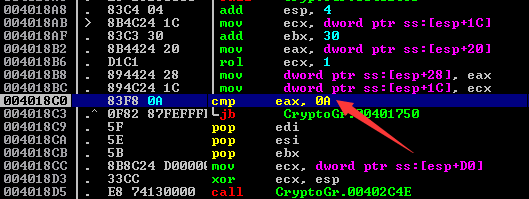Then we can pass the Masterkey 205 to this program and let it run. After a while, I am lucky to get the jpg file which contains the email address:This entry was posted in CTF and tagged , , , . Bookmark the permalink.